Temitayo Oketola | July 18, 2022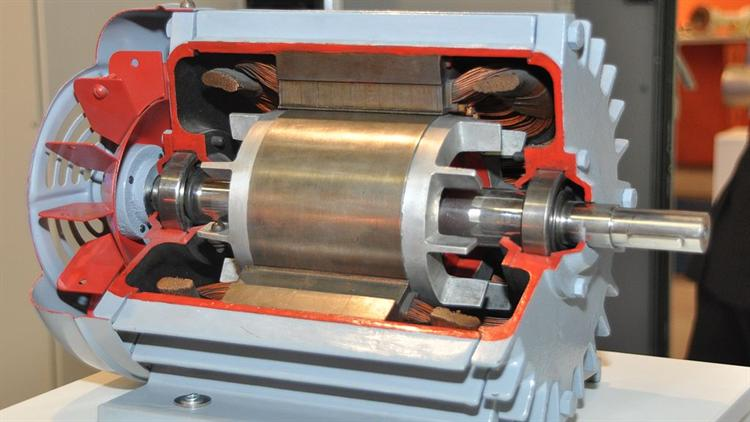Torque slip characteristics help engineers understand how induction motors work under different operating conditions. Source: S.J. de Waard/ CC BY-SA 3.0

Induction motors offer several advantages, allowing them to meet the requirements of various applications. For instance, they have high efficiencies of up to 97%, good speed regulation, overload capacity and high starting torque (the torque the motor generates at zero speed).

However, AC induction motors are not true constant-speed motors because of what engineers call “slip.” In addition, these motors behave differently under different torque and slip values. The torque-slip characteristics help engineers understand how induction motors work under different operating conditions.

## Understanding slip in induction motors

To better understand the slip in induction motors, it is essential to understand how induction motors work.

Induction motors work on the principle of electromagnetic induction. When an induction motor is connected to a three-phase power supply, it causes a rotating magnetic field to be produced in the stator winding. The speed of this rotating magnetic field is called the synchronous speed, and it can be estimated using: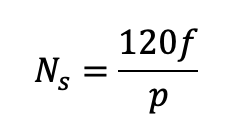Induction motor synchronous speed equation.

Where:

F = incoming line frequency

P = number of stator poles

This rotating magnetic field interacts with the conductors of the rotor, causing EMF (electromotive force) and electromagnetic torque to be induced in the rotor. Therefore, the rotor starts rotating in the same direction as the rotating magnetic field. However, the motor’s rotational speed (or output speed) is usually lesser than its synchronous speed. The difference between the output and synchronous motor speeds is called the slip (s).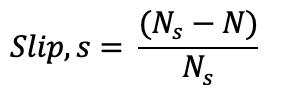Induction motor slip equation.

The torque of the induction motor is related to the slip according to the equation below: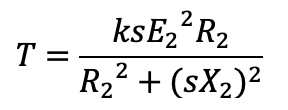Induction motor torque vs. slip equation.

Where:

E2 = rotor induced EMF

s = slip

X2 = rotor inductive reactance

Ns = synchronous speed

R2 = rotor resistance

K = constant of proportionality = (3 x 60)/2πNs

From the above equation, it would be observed that the operation of the motor will be impossible in the absence of slip. In simple terms, the motor torque would be zero if the slip is zero. Likewise, the motor behaves differently when the slip increases or decreases. This motor behavior can be predicted using the torque-slip characteristics of induction motors.

## The torque-slip characteristics of induction motors

The torque-slip characteristics of induction motors can be categorized into three regions:

1. Low slip region
2. Medium slip region
3. High slip region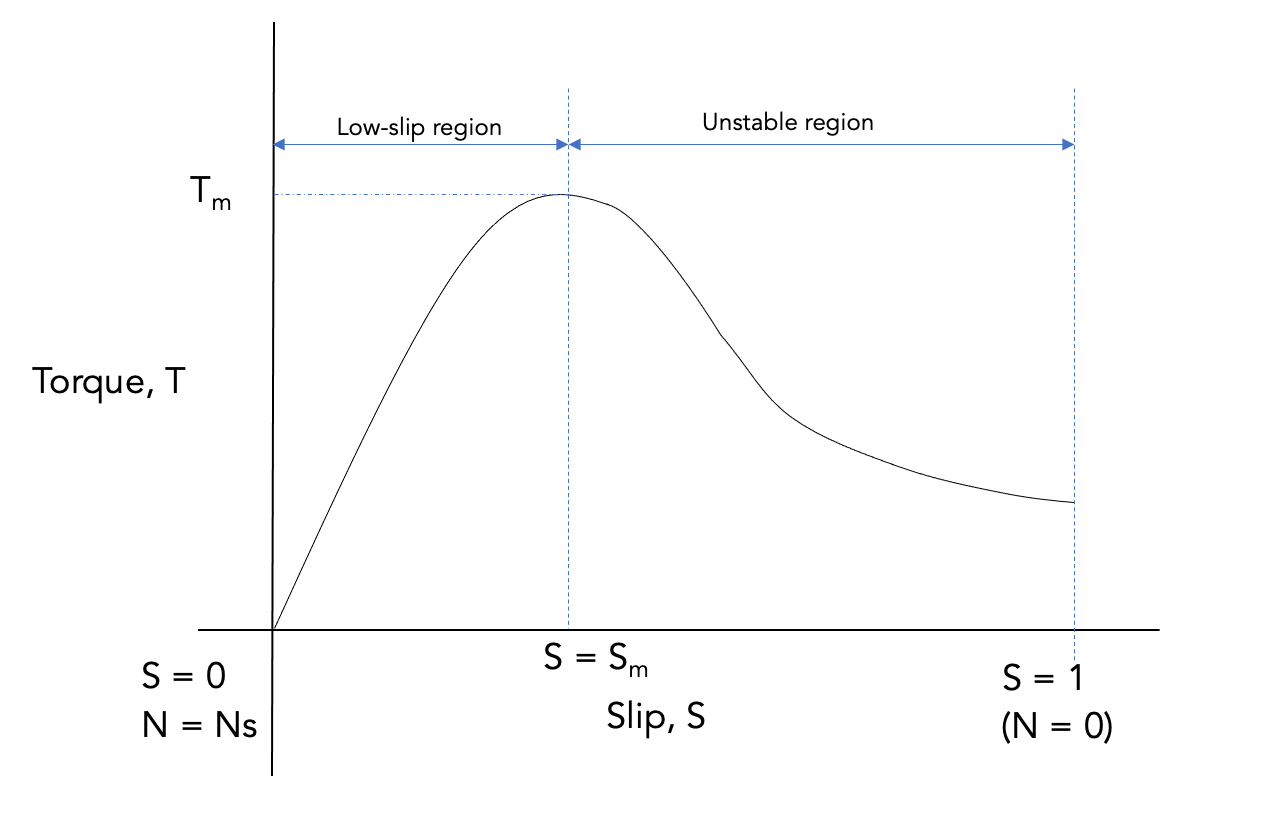Chart 1: Torque-slip characteristics of an induction motor.

### Low slip region

Consider a situation where the rotor speed is near the synchronous speed. In such a scenario, the slip is very low, so the value of (sX2)2 in the torque equation is very small compared to the (R2)2 value. As a result, the (sX2)2 quantity can be neglected, reducing the torque equation to: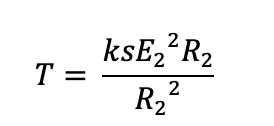Induction motor low slip equation.

Suppose all the quantities in this equation are constant except the slip. In such a scenario, it can be observed that the torque is directly proportional to the slip, as shown below: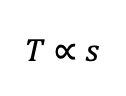Induction motor torque vs. low-slip equation.

Therefore, in the low slip region, the torque increases with the slip. This is the normal operating region for an induction motor, and its torque-slip curve is a straight line.

### Medium slip region

Consider a scenario where the load on the motor increases. In such a scenario, the speed of the motor reduces, causing the slip to increase and the motor to attain its maximum torque value (break down torque or pull out torque). As a result, the (sX2)2 quantity in the torque equation becomes very large compared to the rotor resistance (R2)2. Therefore, the rotor resistance value becomes negligible, reducing the torque equation to: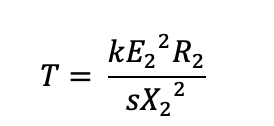Induction motor medium-slip equation.

Since all quantities in this equation are constant except the slip, it will be observed that the torque is inversely proportional to slip, as shown below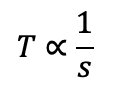Induction motor medium-slip vs. torque equation.

Therefore, at high slip values, an induction motor’s torque-slip curve is shaped like a rectangular hyperbola and passes through the point of maximum torque (Tm).

### High slip region

If the slip continues to increase due to an increase in load beyond the maximum torque, engineers will observe that the torque begins to decrease. If this operation continues long enough, the motor will not be able to pick up the load, causing it to stop eventually. In a worst-case scenario, the motor overheats (and gets damaged), especially if there is no overload protection.

## Conclusion

Slip plays an essential role in the operation of induction motors: it is required to produce torque. However, slip is also among the reasons why AC induction motors are not true constant-speed motors. Engineers can control an induction motor’s speed using variable frequency drives (or adjustable speed AC drives).

Engineers are advised to reach out to induction motor suppliers and variable frequency drive suppliers to discuss their application needs.